×

# IAN50017 - Multiphase machines for automotive applications

This interactive application note presents the advantages of multiphase machines and their use in automotive applications. Some basic principles of multiphase machine control theory as well as important parameters of the control MOSFETs are given.

Author: Nandor Bodo, Applications Engineer, Manchester

This interactive application note contains embedded Cloud based simulations to augment the text.

To open the embedded simulation, simply hover over the simulation image. Left click anywhere in the graphic area once the central play button changes in colour. This opens the schematic in the Cloud environment. See the interactive application note tutorial page for more details on how to use the simulations.

See accompanying application note AN50017.

Figure 1. Multiphase machine and two-level inverter drive

Since the converter can be viewed as an interface that decouples the three-phase mains supply from the machine, the number of the machine’s phases is not limited to three anymore. Thus, with the advent of the inverter, the stage for multiphase (more than three-phase) machines (Fig. 1) has also opened.

The types of multiphase machines for variable-speed applications are in principle the same as their three-phase counterparts. There are induction and synchronous multiphase machines, where a synchronous machine may be with permanent magnet excitation, field winding, or of reluctance type .

Multiphase machines inherently produce a lower harmonic content electromagnetic field. As a consequence, the torque ripple is smaller, the machine noise is reduced and efficiency can be higher than the equivalent three-phase machines . The popularity of multiphase machines is mostly because the supplied power can be redistributed across more phases. As a result, the phase currents are reduced and the need for paralleling or series connection of semiconductor devices is often taken away.

An appreciation of the level at which the phase currents are reduced can be gained from Fig. 2. This trend is especially important in low voltage applications, such as hybrid automotive and small electric vehicles, where the voltage is limited and thus the increase of power must rely on the increase of current.

Another advantage is the reduced strain to the DC-link capacitors. Due to the increased switching the size of the DC-link capacitor can be reduced according to Fig. 3.

As capacitors can be as much as a third of the volume of a three-phase drive, this also amounts to a great increase in the power density of the drive. Along with torque increasing modulation methods and better machine iron utilisation, multiphase machines are well suited to applications requiring small sizes, like automotive.

Figure 3. DC-link capacitance for an increasing number of phasesFigure 2. Phase current in p.u. for different number of phases of multiphase machines

Figure 4. Asymmetrical six-phase and nine-phase machine winding distribution

Multiphase machines also have unexploited levels of control. Strictly speaking, only two currents are enough for creating a rotational field and turning the rotor with a force. However, the multiphase machine has much more phase currents which could in theory be used for other purposes. The prevalent mode of utilization of these degrees of freedom is fault tolerance. The usual approach is to have several sets of three-phase windings with their own drivers and possibly own battery source as well, to ensure redundancy. In case of a failure, the faulty set is shut down or disconnected via a circuit protection switch and the motor can still continue its operation with reduced power capacity.

This approach also enables the individual sets of three-phase windings to be displaced by a cleverly chosen angle, making the magnetic flux harmonic content equivalent to that of a machine with a much higher number of phases. Such is the asymmetrical six-phase and asymmetrical nine-phase winding arrangements Fig. 4.

### Applications of multiphase machines

Since the mid 1990s multiphase drives became serious contenders for various applications. These include electric ship propulsion , locomotive traction, electric and hybrid electric vehicles, “more-electric” aircraft, and high-power industrial applications. In the automotive arena, multiphase machines have been used in electric and hybrid vehicle propulsion, integrated and belt starter/generators and electric power steering.

For automotive applications, the motor is integrated with its drive. This effectively reduces the mass and volume of the motor drive system by combining the enclosures of the two. Consequently, this allows for the cooling system to be shared between the motor and drive and power cables between them shortened, if not eliminated. The fault-tolerant nature of the multiphase machines is then exploited by the modular design of the converter.

The five-phase PMSM along with six-phase BLDC and PMSM machines in generator mode can be inspected in Simulation 1.

### Simulation 1.

Simulation 1. Six-phase asymmetrical BLDC

### Designing in a MOSFET into a multiphase drive

Choosing a MOSFET for a three-phase BLDC and PMSM drives has been addressed in IAN50009. The operation principles of the two machine types are also addressed in the same document and will therefore be omitted here. For a more detailed explanation and derivation of the formulas, the reader can consult the same document.

The voltage rating of the MOSFETs should withstand the battery voltage. However, due to the inductive nature of the load and possible ringing of the waveforms, the actual breakdown voltage is chosen to be up to 50% higher. For battery voltages of 48 V, it is customary to select 80 V or 100 V MOSFETs in more safety-critical applications. If efficiency is more important some designers choose  60 V or 70 V MOSFETs, which inherently have lower RDSon.

The current rating of the MOSFETs can be found relatively easily from the drive power requirement. However, it has to be noted that this value is several times lower than the actual MOSFET current rating that is going to be selected. The actual MOSFET will be selected based on its losses. By calculating the conduction and switching losses and applying those to the MOSFET and PCB thermal model, a selection can be made for a MOSFET that has a junction temperature at about 120 °C at maximum load. The MOSFET itself can stay functional until 175 °C however, the surrounding PCB is usually rated to 125 °C.

For both drive topologies the design starts from the required mechanical output power. Considering the efficiency of the design, mostly dependent on the efficiency of the machine, the input power is calculated:

(Eq 1)The efficiency (η) can take a wide range, but for higher power, automotive machines could be 0.8-0.9.

For the PMSM drives, the current can be calculated from the input power from:

(Eq 2)Where n is the number of phases and Pf is the power factor. Pf can be taken as 0.9 for PMSM machines.

Vrms is calculated from the supply dc voltage:

(Eq 3Where Kn depends on the phase number according to the Table 1. For example for three phase case the maximum achievable Vrms is:

(Eq 4n 3 5 7 9 11 13 15 Kn 1.1547 1.0515 1.0257 1.0154 1.0103 1.0073 1.0055

It is worth noting here that most of the time multiphase machines have an odd number of phases. This is so since then all the phases make a magnetic field that is equally distributed around the rotor circumference. For an even number of phases, if the spatial disposition is the same between the phases, two phases create a magnetic field in the same spatial direction. This means that the harmonic content of the magnetic field of the six-phase machine will be equivalent to that of a three-phase machine. Instead, the machines with an even number of phases (usually it’s the multiple of three as well) have some phases positioned at an angle which is different than 360°/n. For example, the asymmetrical six-phase machine is often utilised. It has two sets of three-phase windings 30° degrees apart (rather than a uniform 360°/6=60°) as shown on the left in Fig. 4. This helps the magnetic field harmonics, while maintaining the DC bus enhancement of 15% quoted in Table 1 for three phase machines.

Finally, the maximum current is:

(Eq 5Gathering (Eq 1), (Eq 2) and (Eq 3) in (Eq 5) the full expression for Im is obtained:

(Eq 6For the BLDC drive, it is assumed that it is made up of multiple three-phase windings. For each three-phase set the input power is:

(Eq 7Therefore, the total input power is:

(Eq 8The maximum current is obtained with a 20% margin:

(Eq 9Therefore, the expression for Im for BLDC drives is:

(Eq 10The conduction losses (per device) for the PMSM and BLDC drives are given in equations (Eq 11) and (Eq 12) respectively:

(Eq 11(Eq 12The theoretical derivation of the conduction losses can be found in IAN50009 for three-phase drives. The equations are identical for multiphase drives as the current waveforms are the same shape as well.

To compare with IAN50009 a switch selection is illustrated here for a 1 kW, 20 kHz drive. The conduction losses are aimed to be 1.5% of the input power. The switching losses are estimated at this time as 50% and 20% of the conduction losses for PMSM and BLDC drives, respectively. This allows for an initial estimation of the total losses as the sum of conduction and switching losses. The maximum current can be calculated as in Table I:

Table 2. Switch rating comparison for 1 kW drive
PSMN BLDC Source
Pmeh 1 kW Application requirement
Vbat 48 V Application requirement
Pel 1.11 kW (η = 0.9) 1.17 kW (η = 0.85) (Eq 1)
Vrms 19.51 V 19.59 V IAN50009
Im 14.9 A 14.7 A (wf = 1.2) (Eq 6) and (Eq 10)

This then allows for the estimation of the required RDSon from (Eq 11) and (Eq 12), by:

(Eq 13for PMSM

(Eq 14for BLDC

The per phase current has halved and the number of devices has doubled, compared to the three phase case. However, since the current appears as a square in the denominator, the final value for the permissible RDSon ends up twice higher than in the three-phase counterpart. The result is RDSon = 17 mΩ for both drive topologies compared to the 8 mΩ in the three-phase case.

### Multiphase machine control theory

The control of multiphase PMSM can be realised in a similar way to the control of three-phase machines. The most common control method is the Field Oriented Control (FOC), displayed in Fig. 5. The outer (position and speed) control loops are the same as in the three-phase case. The output of the speed control loop is the machine torque. The torque influences the reference value for iq (iqref) and the reference for id (idref) influences the magnetisation of the machine. The actual values for iq and id are obtained by decoupling (D) and rotational (R) transformations from the measured phase currents. PI controllers provide the vq and vd voltages from the difference between the reference and real values of id and iq. These voltages are then subject to inverse decoupling (iD) and inverse rotational (iR) transformations to obtain the phase voltages. The phase voltages (va, vb, vc, … vn) are then applied to the space vector or carrier-based modulation blocks (PWM) to obtain the values of the pulse widths (pwa, pwb, pwc, … pwn) that are applied to the power devices used in the inverter.Figure 5. Multiphase PMSM Field Oriented Control

The described so far is identical for three phase and multiphase PMSM’s. However, multiphase machines have other currents resulting from the multiphase Decoupling transformations (ix1, iy1, ix2iyn). These are regulated to zero and thus the  voltages (vx1, vy1, vx2vyn) are obtained via corresponding PI regulators. These are again subject to inverse Decoupling transformations to contribute to the output voltages. This is illustrated in Fig. 5 with red colour.

An example of three-phase and multiphase machine control simulation can be inspected in Simulation 2 and Simulation 3. A three-phase BLDC control can be seen in Simulation 4. These three simulations have similar motor settings. The load inertia has been reduced to reduce simulation time. A torque pulse is applied in the positive direction and then in the negative direction and the rotor accelerates accordingly. The six-phase machine accelerates to the highest speed as the per phase currents are the same and it has more phases. It is demonstrated how the transformations and PI regulators interact with the machine to stipulate torque.

In the case of the BLDC, the transformations are discretised by forcing the switches to apply current through the phases that are in front of the rotor magnetic access and thus pulling it forward.

### Simulation 2

Simulation 2. PMSM Motor and ideal drive loop

### Simulation 3

Simulation 3. Six phase PMSM motor and ideal drive loop

### Simulation 4

Simulation 4. BLDC control

The decoupling and inverse decoupling transformations for a multiphase machine with an odd number of phases as well as for the asymmetrical six- and nine-phase machines are given in the Appendix. As the rotational transformations are applied to only two currents they are the same as in the three-phase case.

### Conclusion

This interactive application note describes the advantages of multiphase machines and prompts the reader to their recent, widely expanded usage. A short description of the power MOSFET switch design in is given, relying heavily on the more descriptive design provided in IAN50009. A short description of the control method for multiphase PMSMs is given with emphasis on the difference between three-phase and multiphase machine control. The differences in the control method lays mostly in the transformation matrices, which are provided in the Appendix.

## Appendix

### Decoupling transformation for machines with an odd number of phases

n is the number of phases, while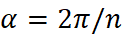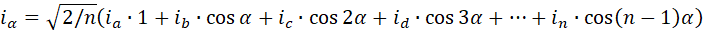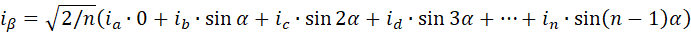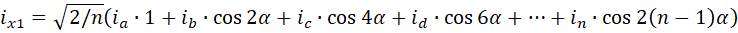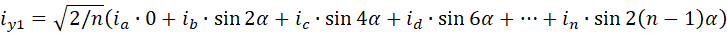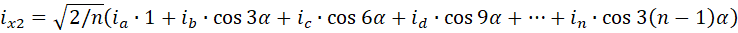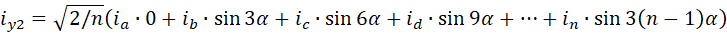…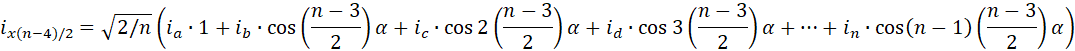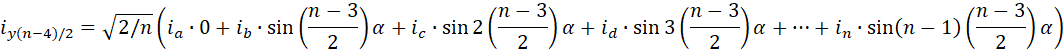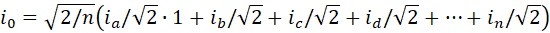### Decoupling transformation for asymmetrical six-phase machines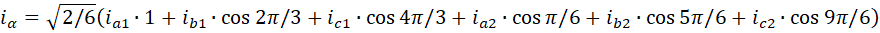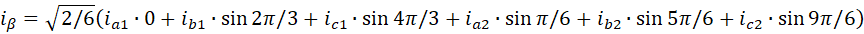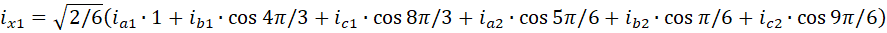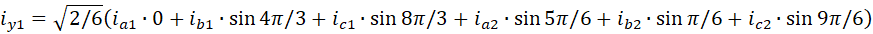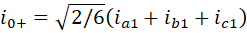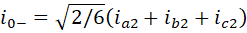### Decoupling transformation for asymmetrical nine-phase machines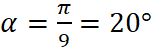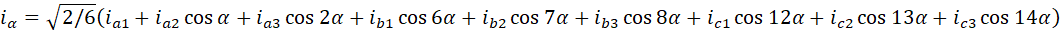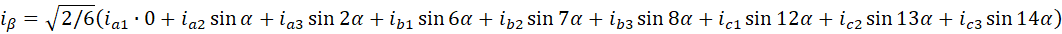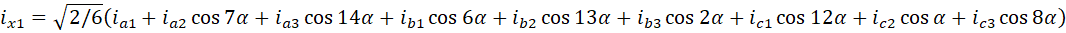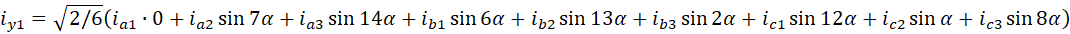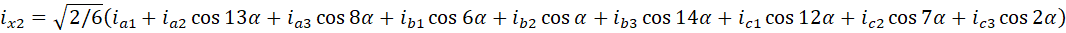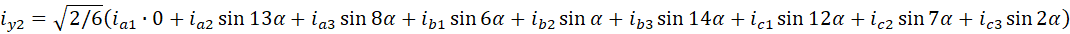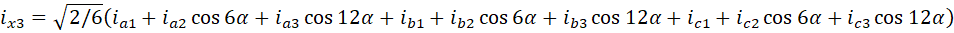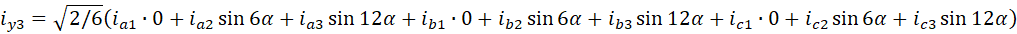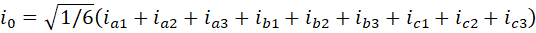### Inverse decoupling transformation for machines with odd number of phases

n is the number of phases, while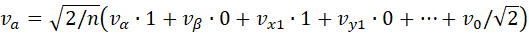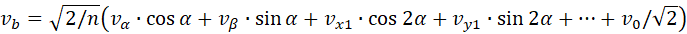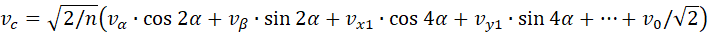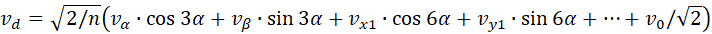…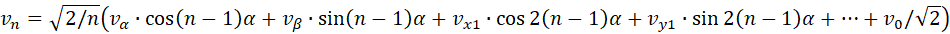### Inverse decoupling transformation for asymmetrical six-phase machines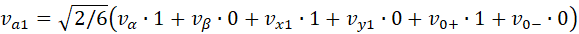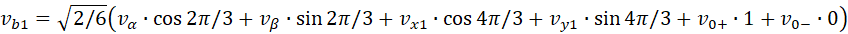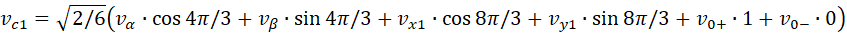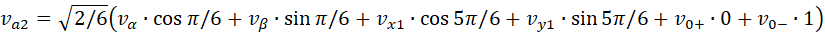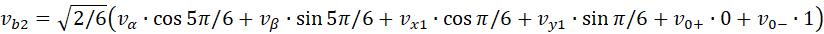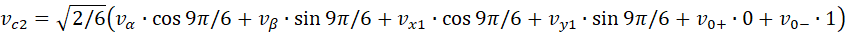### Inverse decoupling transformation for asymmetrical nine-phase machines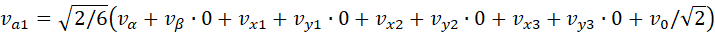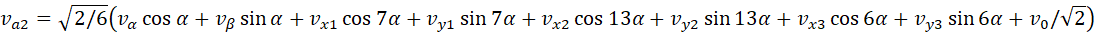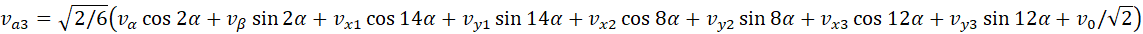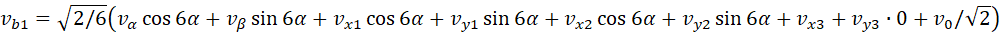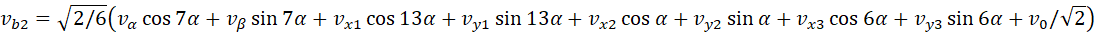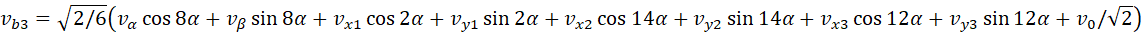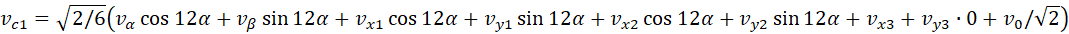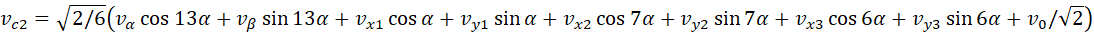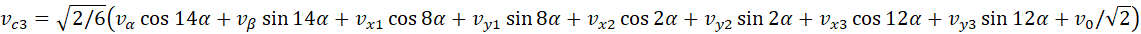### Rotational transformation

θ is the rotor position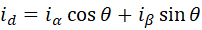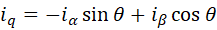### Inverse rotational transformation

θ is the rotor position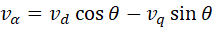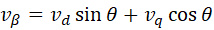### References

1. E. Levi, "Multiphase Electric Machines for Variable-Speed Applications," in IEEE Transactions on Industrial Electronics, vol. 55, no. 5, pp. 1893-1909, May 2008, doi: 10.1109/TIE.2008.918488.

2. C. Hodge, S. Williamson, and A. C. Smith, “Direct drive marine propulsion motors,” in Proc. Int. Conf. Electrical Machines (ICEM), Bruges, Belgium, 2002, CD-ROM, Paper 807.

3. B. M. Wilamowski, and J. D. Irwin, “Power Electronics and Motor Drives”, CRC Press, 2018, ISBN 1439802866, 9781439802861.

 Page last updated 18 July 2022.